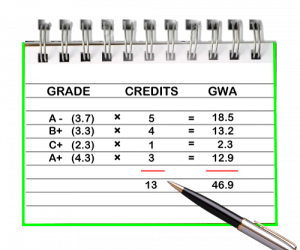Wednesday, May 4, 2022
HomeHow toHow to calculate GPA?

# How to calculate GPA?

GPA is the short form of Grade Point Average. If you are a bachelor’s student, you must be familiar with this term. GPA is a summary of your studies in the institute you study. It is measured semester-wise and can be counted collectively when you complete the degree. GPA is extremely important as it is proof of your academic success. It is not wrong to say that it is the basis of your future. It demonstrates your IQ as well as your behavior towards studies. In the following article, we get to know about How to calculate GPA? So please don’t skip the article from anywhere and read it carefully because it will be very useful for all of you guys.

## Here are the main factors based on which GPA is counted:

• Credit Hours: The sum of time in credits or hours for lectures attended or the overall course.
• Grade points for each grade: For instance, A-grade has 4 points, D grade has 1.0 points, C+ has 2.3 points, and the list goes on.
• Total hours are multiplied by the points assigned to each grade, and as a result, we achieve total grade points.

## Here is How You Can Calculate Your GPA:

Note down the total grade points you earned and multiply them by the total hours attended.

and the total credit hours are 3. Multiply 3 (credit hours) with 3.3 (grade points), and you achieve 9.9 total grade points. Sum and note down hours and grade points of the whole semester. Suppose these count as 12 credit hours and 35.9-grade points. The final step is to divide overall hours(12) with total grade points(35.9), and you will get the result.

So the formula is:

Grade Points ÷ Credit Hours= GPA

So it was the simplest way to count GPA.

## GPA Scale

A GPA scale shows the highest grade average possible for a student to achieve. It represents this average in numerical. Every institute has to select the highest possible grade each student can obtain and choose the weighted option in the weighting prompt of GPA (in the case of weighted GPA scale).

## Types Of GPA Scale

There are two main types of GPA Scales.

1. Unweighted
2. Weighted

The Unweighted Grade Point Average Scale has decided points for each grade. For the quality A point value is 4, 3 points value is for B grade, C has 2 points D has 1 point while the grade F has 0 points.On the other hand, a weighted GPA scale has extra points for every grade level. F has 1 point, D grade has 2 points, C grade has 3 points, B grade has 4 points, and the A grade has 5 points. Well, weighted GPA scales can vary from school to school. For instance, some institutes may assign 5 points to grade A for honors classes but set only 4.5 points for college-level courses; before calculating your GPA, check which type of weighted GPA scale your college uses.

Also read: how to get 1000 followers on Instagram.

RELATED ARTICLES